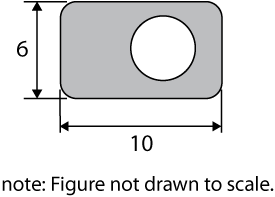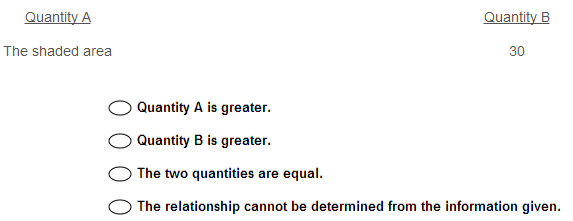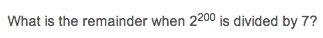Articles tagged "math"

The Math Beast Challenge Problem of the Week – December 2, 2013Each week, we post a new GRE Challenge Problem for you to attempt. If you submit the correct answer, you will be entered into that week’s drawing for two free Manhattan Prep GRE Strategy Guides.

What is the greatest prime factor of 399?

GRE Quantitative Comparison: Don’t Be a Zero, Be a HeroWhen it comes to quantitative comparison questions, zero is a pretty important number, because it’s a weird number. It reacts differently from other numbers when placed in some of the situations. And zero isn’t the only weirdo out there.

Most of us equate “number” with “positive integer”, and for good reason. Most of the numbers we think about and use in daily life are positive integers. Most of our math rules were learned, at least at first, with positive integers.

The GRE knows this, and takes advantage of our assumption. That’s why it’s important to remember all the “other” numbers out there. In particular, when testing numbers to determine the possible values of a variable, there are a few categories of numbers you want to keep in mind.

If I’m going to think about picking numbers, I want to pick numbers that are as different as possible. I try to choose my numbers from a mixture of seven categories, which can be remembered with the word FROZEN:

FR: fractions (both positive and negative)
O: one and negative none
ZE: zero
N: negatives

So we’ve got positive and negative integers (the bigger the absolute value, the better), positive and negative one, positive and negative fractions, and zero. Don’t forget, zero is an integer too!

There are other categories of numbers to think about, particularly if they are mentioned in the problem: odd versus even, prime versus non-prime, etc. But the seven groups listed above account for most of the different ways that numbers behave when you “do math” to them. Because of that fact, picking numbers from different categories can be a fast way to understand the limits of a problem.

To illustrate my point, let’s think about the value of x raised to the power of y. What happens to the value of that expression as y gets bigger? Let’s simplify our lives even further by stipulating that y is a positive integer.

What first comes to mind is the idea that as we increase the value of the exponent, we increase the value of the expression. Well, if x is a positive integer, that’s true: the expression gets exponentially bigger as y increases. Unless x is the positive integer 1, in which case the expression stays the same size, regardless of the value of y. The same is true if x is equal to 0. If x is a positive proper fraction, the expression gets smaller as the value of y increases.

The Math Beast Challenge Problem of the Week – October 21, 2013Each week, we post a new GRE Challenge Problem for you to attempt. If you submit the correct answer, you will be entered into that week’s drawing for two free Manhattan Prep GRE Strategy Guides.The outer figure above is a rectangle with four rounded corners of radius equal to 2.  The inner figure is a circle.Submit your pick over on our Challenge Problem page.

The Math Beast Challenge Problem of the Week – October 7, 2013Each week, we post a new GRE Challenge Problem for you to attempt. If you submit the correct answer, you will be entered into that week’s drawing for two free Manhattan Prep GRE Strategy Guides.See the answer choices and submit your pick over on our Challenge Problem page.

The Math Beast Challenge Problem of the Week – September 30, 2013Each week, we post a new GRE Challenge Problem for you to attempt. If you submit the correct answer, you will be entered into that week’s drawing for two free Manhattan Prep GRE Strategy Guides.

A retailer previously bought an item from a wholesaler for $20 and sold it to consumers for a retail price of$35.  After a wholesale cost reduction, the retailer reduces the retail price by 10%, yet the retailer’s profit on the item still increases by 20%.  By what percent did the wholesale cost decrease?

See the answer choices and submit your pick over on our Challenge Problem page.

GRE Math Refresher or Refreshing Math?During class 2 of our program, we teach a lesson entitled Math Refresher. I thought it would be fun to share one of the problems with you and talk through two things: (1) the actual algebra (real math) we would need to solve the problem, and (2) the standardized testing skills that allow us to solve the problem much more easily.

First, try this problem (© Manhattan Prep). Note: there are no multiple choice answers: you have to come up with a number on your own!

Sarita and Bob together contribute to the cost of a $40 gift. If Bob contributes$12 more than Sarita does, how much does Bob contribute?

How did you do it? Did you write some equations? Try out some numbers? Find some other cool shortcut?

On this one, the best approach is a very neat shortcut that I’m going to show you “ but only after I show you the actual algebra. It’s important to know how to do the algebra, just in case you don’t know or can’t find any good shortcuts.